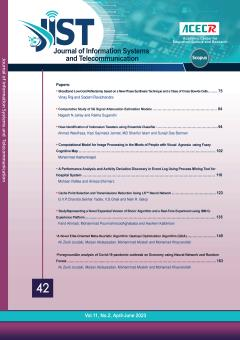• Home
• Babak Haji Bagher Naeeni
• ### Current Issue### Browse

• #### List of ArticlesBabak Haji Bagher Naeeni

• Open Access Article

1 - Achieving Better Performance of S-MMA Algorithm in the OFDM Modulation
Saeed Ghazi-Maghrebi Babak Haji Bagher Naeeni Mojtaba Lotfizad
Effective algorithms in modern digital communication systems provide a fundamental basis for increasing the efficiency of the application networks which are in many cases neither optimized nor very close to their practical limits. Equalizations are one of the preferred More
Effective algorithms in modern digital communication systems provide a fundamental basis for increasing the efficiency of the application networks which are in many cases neither optimized nor very close to their practical limits. Equalizations are one of the preferred methods for increasing the efficiency of application systems such as orthogonal frequency division multiplexing (OFDM). In this paper, we study the possibility of improving the OFDM modulation employing sliced multi-modulus algorithm (S-MMA) equalization. We compare applying the least mean square (LMS), multi modulus algorithm (MMA) and S-MMA equalizations to the per tone equalization in the OFDM modulation. The paper contribution lies in using the S-MMA technique, for weight adaptation, to decreasing the BER in the OFDM multicarrier modulation. For more efficiency, it is assumed that the channel impulse response is longer than the cyclic prefix (CP) length and as a result, the system will be more efficient but at the expense of the high intersymbol interference (ISI) impairment existing. Both analysis and simulations demonstrate better performance of the S-MMA compared to LMS and MMA algorithms, in standard channels with additive white Gaussian noise (AWGN) and ISI impairment simultanously. Therefore, the S-MMA equalization is a good choice for high speed and real-time applications such as OFDM based systems. Manuscript profile
• Open Access Article

2 - Optimization of Random Phase Updating Technique for Effective Reduction in PAPR, Using Discrete Cosine Transform
Babak Haji Bagher Naeeni
One of problems of OFDM systems, is the big value of peak to average power ratio. To reduce it, any attempt have been done amongst which, random phase updating is an important technique. In contrast to paper, since power variance is computable before IFFT block, the com More
One of problems of OFDM systems, is the big value of peak to average power ratio. To reduce it, any attempt have been done amongst which, random phase updating is an important technique. In contrast to paper, since power variance is computable before IFFT block, the complexity of this method would be less than other phase injection methods which could be an important factor. Another interesting capability of random phase updating technique is the possibility of applying the variance of threshold power. The operation of phase injection is repeated till the power variance reaches threshold power variance. However, this may be a considered as a disadvantage for random phase updating technique. The reason is that reaching the mentioned threshold may lead to possible system delay. In this paper, in order to solve the mentioned problem, DCT transform is applied on subcarrier outputs before phase injection. This leads to reduce the number of required carriers for reaching the threshold value which results in reducing system delay accordingly. Manuscript profile